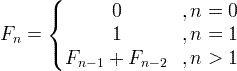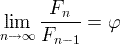# Fibonacci numbers & sequence

Fibonacci sequence is a sequence of numbers, where each number is the sum of the 2 previous numbers, except the first two numbers that are 0 and 1.

## Fibonacci sequence formula#### For example:

F0 = 0

F1 = 1

F2 = F1+F0 = 1+0  = 1

F3 = F2+F1 = 1+1  = 2

F4 = F3+F2 = 2+1  = 3

F5 = F4+F3 = 3+2  = 5

...

## Golden ratio convergence

The ratio of two sequential Fibonacci numbers, converges to the golden ratio:φ is the golden ratio = (1+√5) / 2 ≈ 1.61803399

n Fn
0 0
1 1
2 1
3 2
4 3
5 5
6 8
7 13
8 21
9 34
10 55
11 89
12 144
13 233
14 377
15 610
16 987
17 1597
18 2584
19 4181
20 6765

TBD

## C code of Fibonacci function

double Fibonacci(unsigned int n)

{

double f_n =n;

double f_n1=0.0;

double f_n2=1.0;

if( n > 1 ) {

for(int k=2; k<=n; k++) {

f_n  = f_n1 + f_n2;

f_n2 = f_n1;

f_n1 = f_n;

}

}

return f_n;

}

Currently, we have around 5613 calculators, conversion tables and usefull online tools and software features for students, teaching and teachers, designers and simply for everyone.